×#### Thank you for registering.

One of our academic counsellors will contact you within 1 working day.

Click to Chat

1800-1023-196

+91-120-4616500

CART 0

• 0

MY CART (5)

Use Coupon: CART20 and get 20% off on all online Study Material

ITEM
DETAILS
MRP
DISCOUNT
FINAL PRICE
Total Price: Rs.

There are no items in this cart.
Continue Shopping• Complete JEE Main/Advanced Course and Test Series
• OFFERED PRICE: Rs. 15,900
• View Details

```Chapter 6: Fractions – Exercise 6.6

Question: 1

Reduce each of the following fractions to its lowest term (simplest form):

i)  40/75

ii)  42/28

iii)  12/52

iv)  40/72

v)  80/24

vi)  84/56

Solution:

i)  40/75

Factors of 40 are 1, 2, 4, 5, 8, 10, 20 and 40.

Factors of 75 are 1, 3, 5, 15 and 75.

Common factors of 40 and 75 are 1 and 5.

HCF = 5

Divide both the numerator & denominator by 5.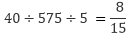Therefore, the simplest form obtained is 8/15

ii)  42/28

Factors of 42 are 1, 2, 3, 6, 7, 14, 21, 42

Factors of 28 are 1, 2, 4, 7, 14, 28

Common factors of 42 & 28 are 1, 2 and 4.

HCF = 4

Divide both the numerator & denominator by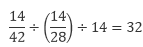Therefore, the simplest form obtained is 32

iii)  12/52

Factors of 12 are 1, 2, 3, 4, 6 and 12.

Factors of 52 are 1, 2, 4, 13, 26 and 52.

Common factors of 12 and 52 are 1, 2 and 4

HCF = 4

Divide both the numerator & denominator by 4.

On solving the above we have,

3/13

Therefore, the simplest form obtained is 3/13

iv) 40/72

Factors of 40 are 1, 2, 4, 5, 8, 10, 20, 40

Factors of 72 are 1, 2, 3, 4, 6, 8, 9, 12, 18, 24, 36, 72

Common factors of 40 and 72 are 1, 2, 4 and 8.

HCF = 8

Divide both the numerator & denominator by 8.

On solving the above equation, we have:

5/9

Therefore, the simplest form obtained is 5/9

v) 80/24

Factors of 80 are 1, 2, 4, 5, 8, 10, 16, 20, 40 and 80.

Factors of 24 are 1, 2, 3, 4, 6, 8, 12 and 24.

Common factors of 80 and 24 are 1, 2 , 4 , 8

HCF = 8

Divide both the numerator & denominator by 4.

On solving the above equation, we have:

10/3

Therefore, the simplest form obtained is 10/3

vi) 84/56

Factors of 84 are 1, 2, 3, 4, 6, 7, 12, 14, 21, 28, 42 and 84.

Factors of 56 are 1, 2, 4, 7, 8, 14, 28 and Common factors of 84 & 56 are 1, 2, 4, 7, 14 and 28.

HCF = 28

Divide both the numerator & denominator by 28

On solving the above equation, we have:

3/2

Therefore, the simplest form obtained is 3/2

Question: 2

Simplify each of the following to its lowest form:

i)  75/80

ii)  52/76

iii)  84/98

iv)  68/17

v)  150/50

vi)  162/108

Solution:

i) 75/80

Factors of 75 are 1, 3, 5, 15, 25 and 75.

Factors of 80 are 1, 2, 4, 5, 8, 10, 12, 16, 20, 40 and 80.

Common factors of 75 and 80 are 1 and 5.

HCF of 75 and 80 is 5.

Dividing both the numerator and denominator by 5, we get: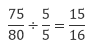Therefore, the simplest form obtained is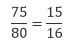ii)  52/76

Factors of 52 are 1, 2, 4, 13, 26 and 52.

Factors of 76 are 1, 2, 4, 19, 38 and 76.

Common factors of 52 and 76 are 1, 2 and 4.

HCF of 52 and 76 is 4.

Dividing both the numerator and denominator by 5, we get: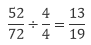Therefore, the simplest form obtained is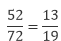iii)  84/98

Factors of 84 are 1, 2, 3, 4, 6, 7, 12, 14, 21, 28, 42 and 84.

Factors of 98 are 1, 2, 7, 14, 49 and 98.

Common factors of 84 and 98 are 1, 2, 7 and 14.

HCF of 84 and 98 is 14

Dividing both the numerator and denominator by 5, we get:Therefore, the simplest form obtained is

84/98 = 6/7

iv) 68/17

Factors of 68 are 1, 2, 4, 17, 34 and 68.

Factors of 17 are 1 and 17.

Common factor of 68 and 17 is 17

HCF of 68 and 17 is 17

Dividing both the numerator and denominator by 5, we get: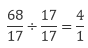Therefore, the simplest form obtained is

68/17 = 4/1

v) 150/50

Factors of 150 are 1, 2, 3, 5, 6, 10, 15, 25, 50 and 150.

Factors of 50 are 1, 2, 5, 10, 25 and 50.

Common factor of 150 and 50 is 50

HCF of 150 and 50 is 50

Dividing both the numerator and denominator by 50, we get:Therefore, the simplest form obtained is

150/50 = 3/1

vi) 162/108

Factors of 162 are 1, 2, 3, 6, 9, 18, 27, 54, 81 and 162.

Factors of 108 are 108, 1, 2, 3, 4, 6, 9, 12, 18, 27 and 54.

Common factor of 162 and 108 are 1, 2, 3, 6, 9, 18, 27, 54

HCF of 162 and 108 is 54

Dividing both the numerator and denominator by 54, we get:Therefore, the simplest form obtained is

162/108 = 3/2
```### Course Features

• 728 Video Lectures
• Revision Notes
• Previous Year Papers
• Mind Map
• Study Planner
• NCERT Solutions
• Discussion Forum
• Test paper with Video Solution